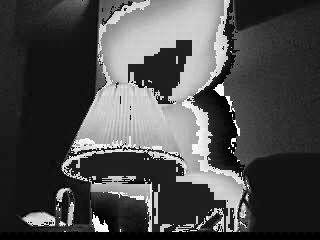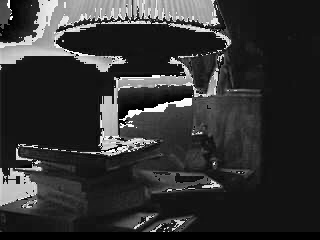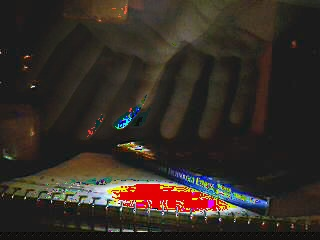# Screwy OpenCV Manipulations

## March 2, 2008

I’ve been playing more with OpenCV and I think I’m missing something. I can’t do any manipulations on the image data without really screwing it up. The only thing that doesn’t seem to wash out the data out is moving pixels around without changing them. Not sure what I’m missing. Here’s the few different manipulations and what they look like when they wash out.

Monochrome

```for(int i = 0; i < frame->height; i++) {
int offset = i*frame->width*3;
for(int j = 0; j < frame->width; j++) {
uchar temp = frame->imageData[offset+(j*3)]*0.114
+ frame->imageData[offset+(j*3)+1]*0.587
+ frame->imageData[offset+(j*3)+2]*0.299;
frame->imageData[offset+(j*3)] = temp;
frame->imageData[offset+(j*3)+1] = temp;
frame->imageData[offset+(j*3)+2] = temp;
}
}```Memory
This one just keeps five frames and then adds them in to create a faded composite, should be simple.

```if(0 == memory_frameCounter)
memory_frames = cvCloneImage(frame);
else if(2 == memory_frameCounter)
memory_frames = cvCloneImage(frame);
else if(4 == memory_frameCounter)
memory_frames = cvCloneImage(frame);
else if(6 == memory_frameCounter)
memory_frames = cvCloneImage(frame);
else if(8 == memory_frameCounter)
memory_frames = cvCloneImage(frame);
else if(10 <= memory_frameCounter)
memory_frameCounter = -1;

memory_frameCounter++;

for(int i = 0; i < frame->height; i++) {
for(int j =0; j < frame->width*3; j++) {
memory_agg = frame->imageData[(i*frame->width*3)+j];
for(int k = 0; k < 5; k++) {
memory_agg = (memory_agg + memory_frames[k]->imageData[(i*frame->width*3)+j])/2;
}
frame->imageData[(i*frame->width*3)+j] = memory_agg;
}
}```I just can’t figure out what I’m doing wrong here.

1. […] I have some code and images here: http://www.velvetcache.org/2008/03/02/screwy-opencv-manipulations […]

2.giulio says:

try using cvSet2D and cvGet2D, or using img->widthstep instead of (img->width)*3.. often it’s not the same

```IplImage* img = cvLoadImage(.....); //your image
int width = img->width;
int height = img->height;
for (int j=0; j < height; j++){
for (int i=0; i < width; i++){

CvScalar rgb = cvGet2D(img, j, i);
int gray = rgb*0.114 + rgb*0.587 +
rgb*0.299;
}
}
```
3.john says:

Works like a charm! Here’s the code I ended up with for it.

```int width = frame->width;
int height = frame->height;
for (int j=0; j < height; j++){
for (int i=0; i < width; i++){
rgb = cvGet2D(frame, j, i);
int gray = rgb.val*0.114 + rgb.val*0.587 +
rgb.val*0.299;
rgb.val = gray;
rgb.val = gray;
rgb.val = gray;
cvSet2D(frame,j,i,rgb);
}
}```
4. […] That’s all over now thanks to the OpenCV mailing list, you can see the fix in the comments of this post. Since I can now manipulate colors and pixels with ease I’ve been writing a few more neat […]